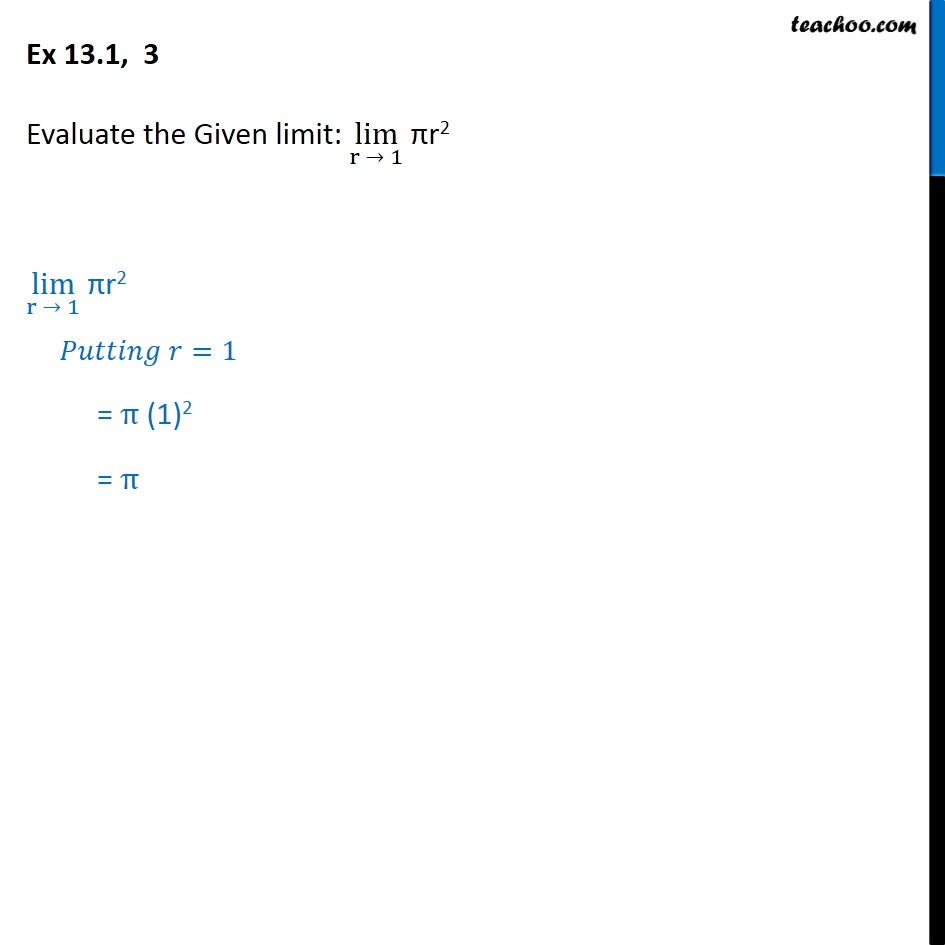1. Chapter 13 Class 11 Limits and Derivatives (Term 1 and Term 2)
2. Serial order wise
3. Ex 13.1 (Term 1)

Transcript

Ex 13.1, 3 - Chapter 13 Class 11 Limits and Derivatives - NCERT Evaluate the Given limit: lim (r → 1) πr^2 To find the limit, first we put value of r and see if its defined or not lim (r → 1) πr^2 Putting r = 1 = π (1)^2 = π